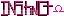-=+=- -=+=- -=+=- -=+=- -=+=- -=+=- -=+=- -=+=- -=+=- -=+=- -=+=- -=+=- -=+=- -=+=- -=+=- -=+=- -=+=- -=+=- -=+=- -=+=- -=+=- -=+=- -=+=- -=+=- -=+=- -=+=- -=+=- -=+=- -=+=- -=+=- (c) WidthPadding Industries 1987 0|308|0 -=+=- -=+=- -=+=- -=+=- -=+=- -=+=- -=+=- -=+=- -=+=- -=+=- -=+=- -=+=- -=+=- -=+=- -=+=- -=+=- -=+=- -=+=- -=+=- -=+=- -=+=- -=+=- -=+=- -=+=- -=+=- -=+=- -=+=- -=+=- -=+=- -=+=-
SoCoder -> Blogs Home -> Blogs

blanko1324Created : 07 July 2008

### Swaying Trees

Still messing around with my "Nice Little Map System", I decided to replace the marker trees with something a little less...static.Each of the colored bands sway individually. The images were made out of construction paper. If you would like to see a demo, download here. The demo generates a random forest.

Monday, 07 July 2008, 14:47
JayenkaiNeat!

A little aliased, though.. Maybe due to the teensy rotations.. Perhaps a simple left-right movement might help reduce the amount of aliasing..

But otherwise..

Mmmmm!!!
Can't wait to see the main chr if the trees look that great!Monday, 07 July 2008, 15:21
blanko1324Yeah, the aliasing is just something I have to put up with using the graphics engine. There's an optional texture filter which solves the problem, but just creates an uglier mess, shown here in the outlines:I'll try out your idea though, and see how that does.Monday, 07 July 2008, 19:11
Schererererneat! is everything going to be made out of construction paper in this game? kinda like how platypus was done with clay~~ you could do an origami thing. looking forward to more demos!Wednesday, 09 July 2008, 00:02
blanko1324I really haven't decided yet. Most likely, it'll end up being multiple styles. Maybe some marker here, crayon there, construction paper, tin foil, whatever...Wednesday, 09 July 2008, 11:04
PhoenixCreativeI wouldn't have thought of moving them like that to create animation. I'm looking forward to see whatever this turns into.
Wednesday, 09 July 2008, 13:03
Orion PaxThis is really good blanko. I like the construction paper look to it. I hope you will even release your map system as a userlib. I would try and make an rpg game with this.